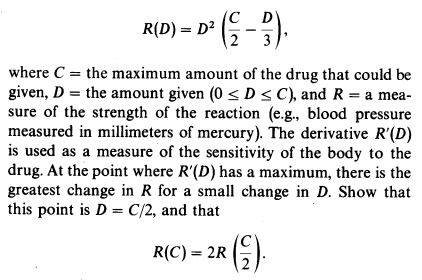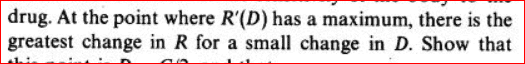# Min max: optimal quantity of medicine

• Karol

## Homework Statement## Homework Equations

Minimum/Maximum occurs when the first derivative=0

## The Attempt at a Solution

$$R'=2D\left( \frac{C}{2}-\frac{D}{3} \right)-\frac{1}{3}D^2$$
$$R'=0~\rightarrow~D=C$$

Last edited:

## Homework Statement

View attachment 230882

## Homework Equations

Minimum/Maximum occurs when the first derivative=0

## The Attempt at a Solution

$$R'=2D\left( \frac{C}{2}-\frac{D}{3} \right)-\frac{1}{3}D^2$$
$$R'=0~\rightarrow~D=C$$It discusses the point where ##\ R^\prime (D) \ ## is a maximum, not about the max of ## R(D)\,.##

Also, it may help to write R(D) as: ##\ \displaystyle R(D) = \frac{D^2C}{2}-\frac{D^3}{3} \,.##

.

#### Attachments

$$R'=2D\left( \frac{C}{2}-\frac{D}{3} \right)-\frac{1}{3}D^2,~~R''=C-2D,~~R''=0:~D=\frac{C}{2}$$
But the greatest change in R for a small change in D is where R has a maximum, hence where R'=0, not where R''=0

$$R'=2D\left( \frac{C}{2}-\frac{D}{3} \right)-\frac{1}{3}D^2,~~R''=C-2D,~~R''=0:~D=\frac{C}{2}$$
But the greatest change in R for a small change in D is where R has a maximum, hence where R'=0, not where R''=0
Read the problem again. It's R'(D) which you need to find the maximum for, not finding the maximum for R(D) .

Yes, that's correct, i need the maximum for R', but why?
At the point where R has a maximum, i think, a small change in D makes a big change in R

Yes, that's correct, i need the maximum for R', but why?
At the point where R has a maximum, i think, a small change in D makes a big change in R

No, that's where R' is a maximum. R' = 0 is where there is no change with a change in D.

Yes, that's correct, i need the maximum for R', but why?
At the point where R has a maximum, i think, a small change in D makes a big change in R

No: at the maximum a small change in ##D## makes NO change in ##R##! The tangent line to the graph ##R = f(D)## is horizontal at an interior maximum; that is why we look for points where the derivative vanishes when maximizing or minimizing.

You really need to have a better intuitive understanding of this material, and to help with that I suggest that you substitute some numerical value for ##C##, then plot ##R(D)## and ##R'(D)##.

•SammyS
Thanks